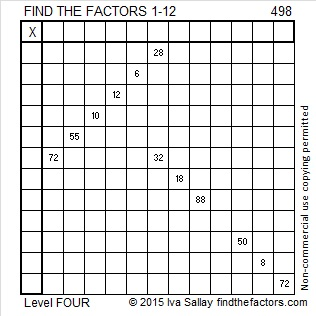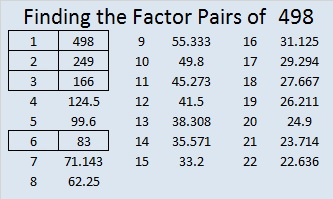# 498 and Level 4

As I searched for something interesting yet understandable to say about the number 498, I learned that 498 is the third 167-gonal number when I looked at virtuescience.com/polygonal-numbers. I also found out that “Charlie Eppes” has a blog called Numbers that features graphic representations of the number 498 and a lot of other numbers, too.

You may find puzzle #498 to be a little trickier than most Level 4 puzzles, but I know you can still meet the challenge.Print the puzzles or type the solution on this excel file: 12 Factors 2015-05-18

—————————————————————————————————

• 498 is a composite number.
• Prime factorization: 498 = 2 x 3 x 83
• The exponents in the prime factorization are 1, 1, and 1. Adding one to each and multiplying we get (1 + 1)(1 + 1)(1 + 1) = 2 x 2 x 2 = 8. Therefore 498 has exactly 8 factors.
• Factors of 498: 1, 2, 3, 6, 83, 166, 249, 498
• Factor pairs: 498 = 1 x 498, 2 x 249, 3 x 166, or 6 x 83
• 498 has no square factors that allow its square root to be simplified. √498 ≈ 22.3159136—————————————————————————————————